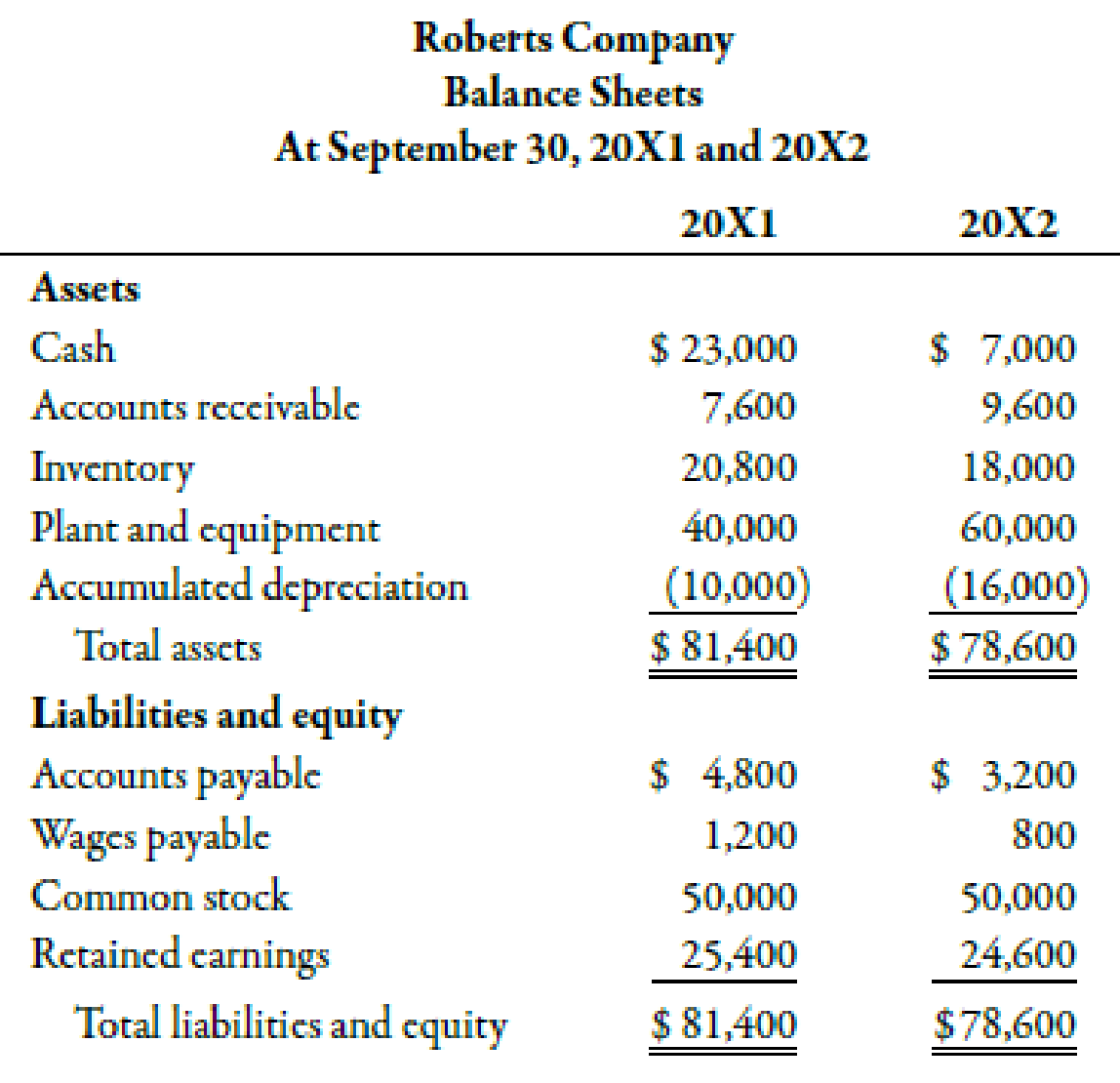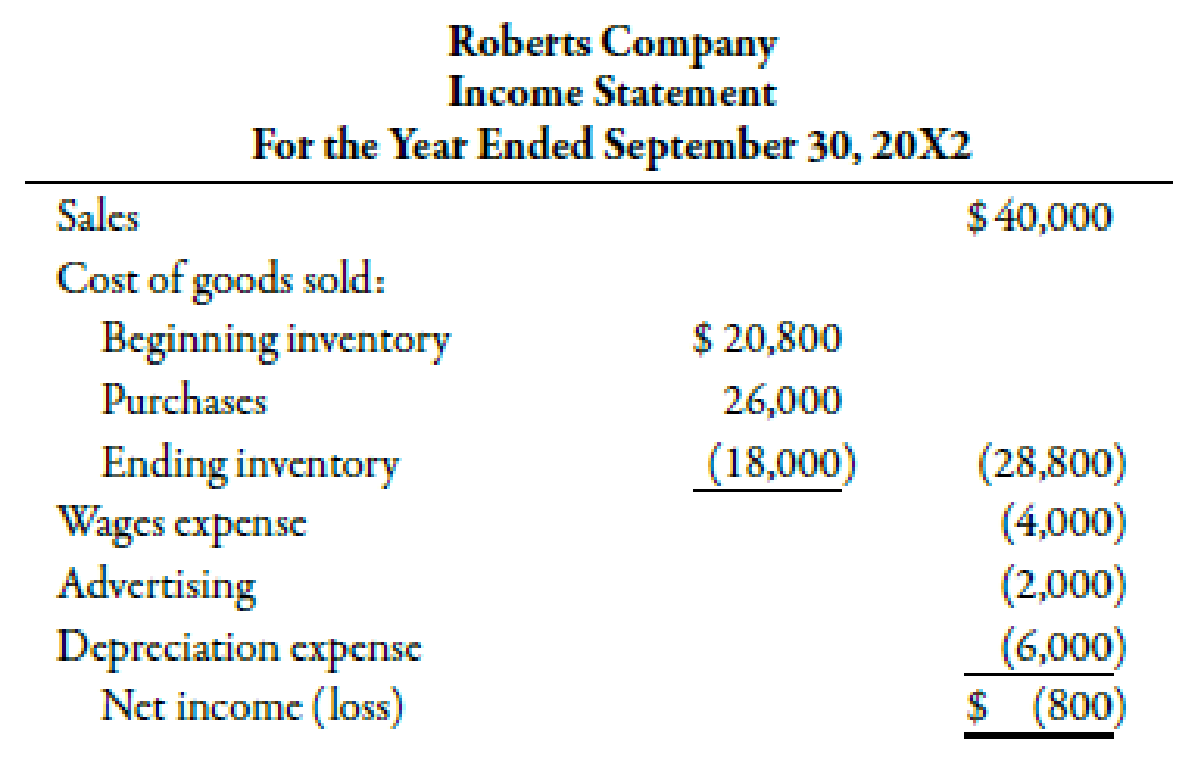Chapter 14, Problem 47P### Managerial Accounting: The Corners...

7th Edition
Maryanne M. Mowen + 2 others
ISBN: 9781337115773

#### Solutions

Chapter
Section### Managerial Accounting: The Corners...

7th Edition
Maryanne M. Mowen + 2 others
ISBN: 9781337115773
Textbook Problem
27 views

# The following financial statements were provided by Roberts Company:At the end of 20X2, Roberts purchased some additional equipment for $20,000.Required:Calculate operating cash flows using the direct method. To determine Chart the net operating cash flows using the direct method. Explanation Cash Flows from Operating Activities: This category of a cash flow statement shows the operational and profit generating activities in a firm. The operating cash flows increase or decrease the current assets and current liabilities of a firm. The cash flows from operating activities for R Company for 20X2 using direct method are shown below:  R Company Statement of Cash Flow For the year ended September 30, 20X2 Particulars Income statement ($) Adjustments ($) Cash flows ($) Cash flows from operating activities: Revenues 40,000 (2,000)1 38,000 Cost of goods sold (28,800) (2,800)2 - (1,600)3 (27,600) Wages expenses (4,000) 4004 (4,400) Advertising (2,000) (2,000) Depreciation expense (6,000) 6,0005 - Net cash from operating activities 4,000

Table (1)

Therefore, the net cash from operating activities is $4,000. Working Note: 1. Calculation of difference in accounts receivable: (DifferenceinAccountsReceivable)=(AmountofAccountsReceivableof20X2AmountofAccountsReceivableof20X1)=($9,600$7,600)=$2,000

2.

Calculation of difference in amount of inventories:

(DifferenceinInventories)=(AmountofInventoriesof20X2AmountofInventoriesof20X1)=($18,000$20,800)=\$2,800

3

### Still sussing out bartleby?

Check out a sample textbook solution.

See a sample solution

#### The Solution to Your Study Problems

Bartleby provides explanations to thousands of textbook problems written by our experts, many with advanced degrees!

Get Started